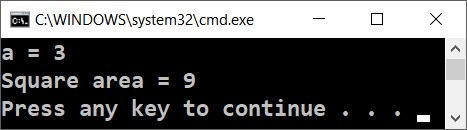Example: Calculating a Square Area

For example, let us look at the following program, which reads an integer from the console, multiplies it by itself (squares it) and prints the result from the multiplication.

Video: Calculating a Square Area

Watch a video lesson about calculating square area: https://youtu.be/gdYTotTFVgA.

Code: Calculating a Square Area

This code demonstrates how we can calculate the square area by the given length of the side:

Console.Write("a = ");
var area = a * a;
Console.Write("Square area = ");
Console.WriteLine(area);


Here is how the program would work when we have a square with a side length equal to 3:Try to write a wrong number, for example "hello". You will get an error message during runtime (exception). This is normal. Later on, we will find out how we can catch these kinds of errors and make the user enter a number again.

How Does the Example Work?

The first line Console.Write("a = "); prints an informative message, which invites the user to enter the side of the square a. After the output is printed, the cursor stays on the same line. Staying on the same line is more convenient for the user, visually. We use Console.Write(…), and not Console.WriteLine(…) and this way the cursor stays on the same line.

The next line var a = int.Parse(Console.ReadLine()); reads an integer from the console. Actually, it first reads a text (string) using Console.ReadLine() and after that it gets converted to an integer (it is parsed) using int.Parse(…). The result is kept in a variable with name a.

The next command var area = a * a; keeps in a new variable area the result of the multiplication of a by a.

The next command Console.Write("Square area = "); prints the given text without going to the next line. Again, use Console.Write(…), and not Console.WriteLine(…), and this way the cursor stays on the same line in order to print the calculated area of the square afterwards.

The last command Console.WriteLine(area); prints the calculated value of the variable area.